# Part 6: Volume

In this article, we develop the skills you learned while studying surface area and apply them to calculating the volume of shapes.## Volume

Being able to determine the volume of composite solids is an essential skill that is necessary for several Year 11 and Year 12 topics such as optimisation. It is important that you understand the meaning of each term in the volume formulas now because it will be useful in the long run. This will become assumed knowledge in the years ahead!

## NSW Syllabus Outcomes

This article addresses the following syllabus outcomes:

• Stage 5.2: Solve problems involving the volumes of right prisms (ACMMG218)
• Find the volumes of composite right prisms with cross-sections that may be dissected into triangles and special quadrilaterals.
• Solve a variety of practical problems related to the volumes and capacities of composite right prisms
• Stage 5.3: Solve problems involving the volumes of right pyramids, right cones, spheres and related composite solids (ACMMG271)
• Develop and use the formula to find the volumes of right pyramids and right cones
• Find the volume of spheres and composite solids that include right pyramids, right cones and hemispheres.

These Outcomes will, like Surface Areas, equip you to be able to evaluate the volumes of real-world objects so you can discuss them accurately.

In addition, to the cylinders, cones, and spheres we looked at in the previous article, we shall also be looking at how to calculate the volume of prisms.

## Assumed Knowledge

Students should be familiar with the conversion between units of volume as well as conversion between units of length: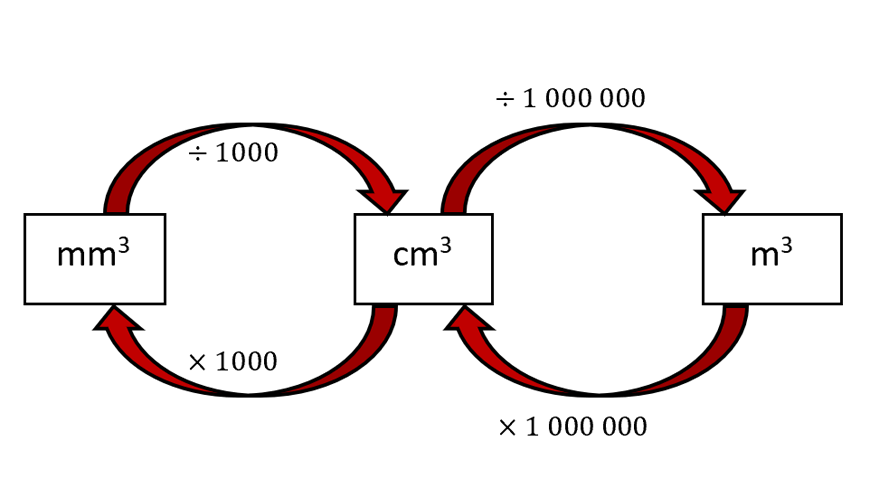Conversion of Volume Units

Knowledge of how to determine the area of composite shapes that may be broken down into special quadrilaterals, triangles and circles/semicircles will also be required.

## Prisms

A prism is defined as a solid geometric figure that has the same plane shape for its cross-sectional face across its entire height. Examples of prisms are shown below: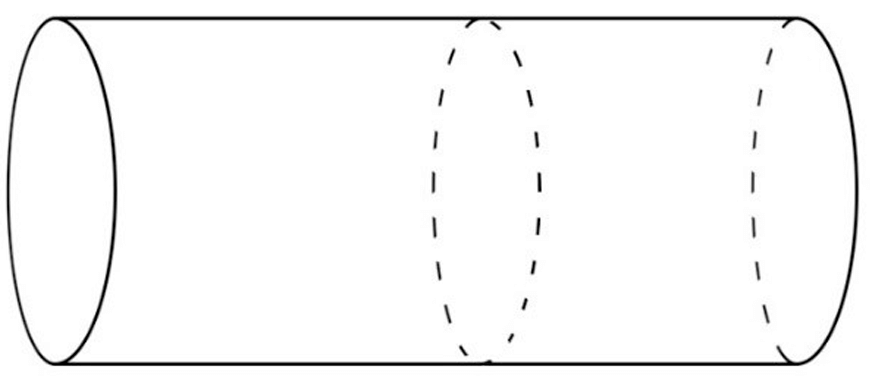Cylindrical prism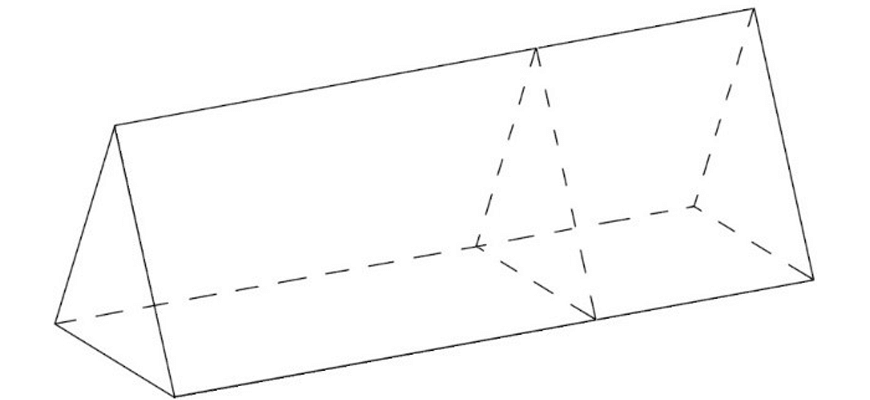Triangular prism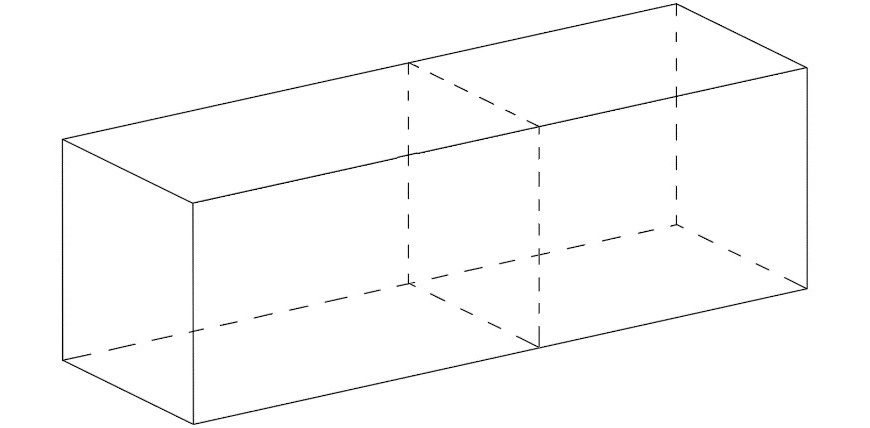Square Prism

When we need to determine the volume of a prism, we use the formula:

$$V_{\text{prism}} = A_{\text{base}} \times h$$

Where:

• $$V_{\text{prism}}$$ is the volume of the prism
• $$A_{\text{base}}$$ the area of the base of the prism
• $$h$$ the distance between the congruent faces of the prism (the height of the prism)

For the three prisms shown above, their bases have been shaded-in and their heights have been marked below.

Note that the base of the prism is the side which has a plane shape that is congruent to any cross sections taken in the same direction: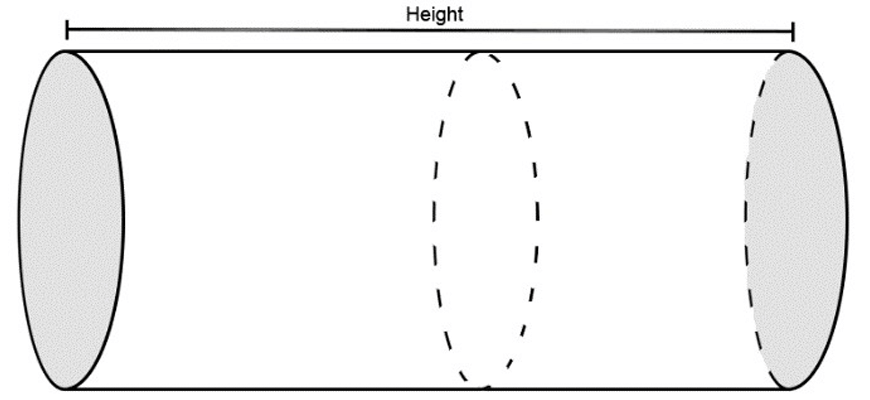Shaded cylindrical prism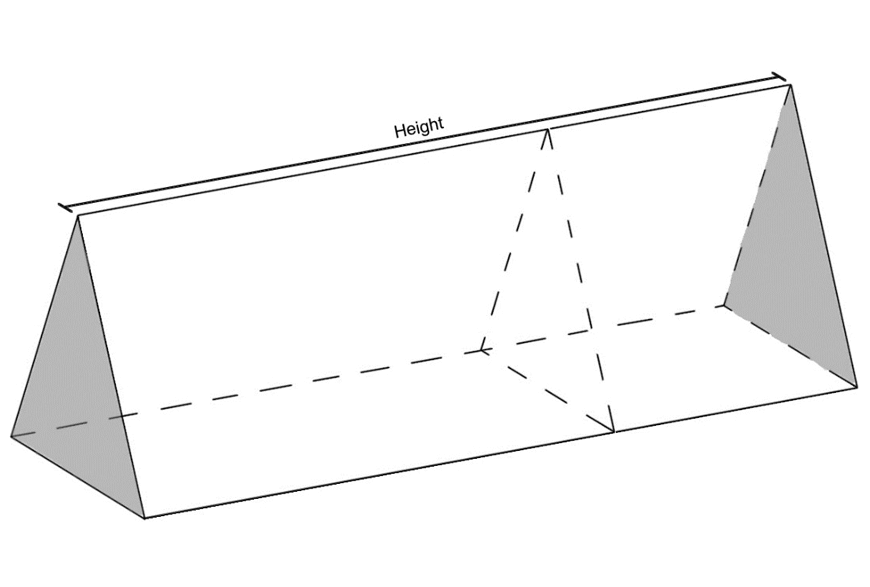Shaded triangular prism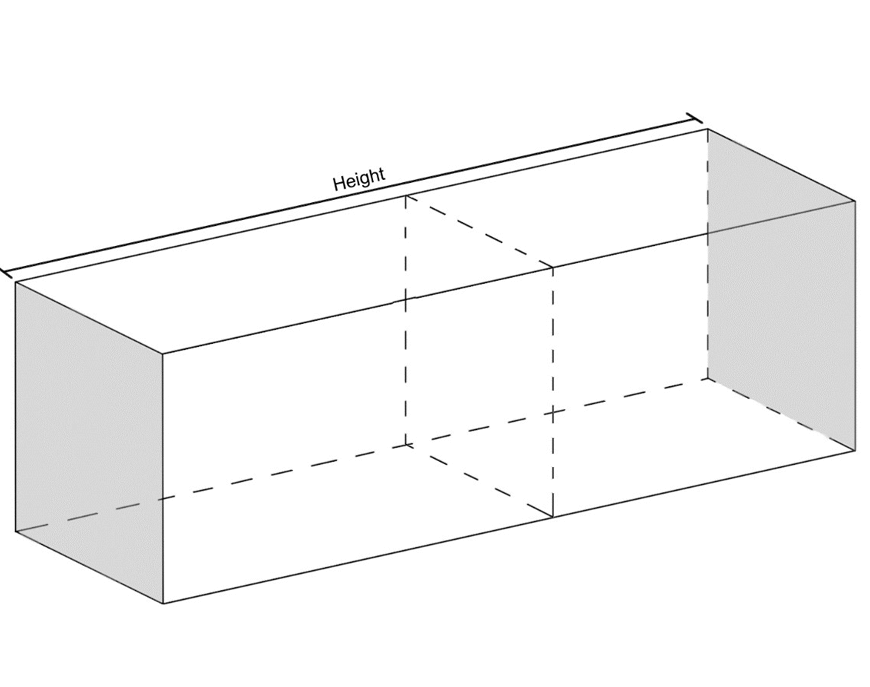Shaded square prism

Notice how the area of this prism’s base is identical to the area of its uniform cross section.

### Example:

Determine the volume of the trapezoidal prism below. The diagram is not to scale: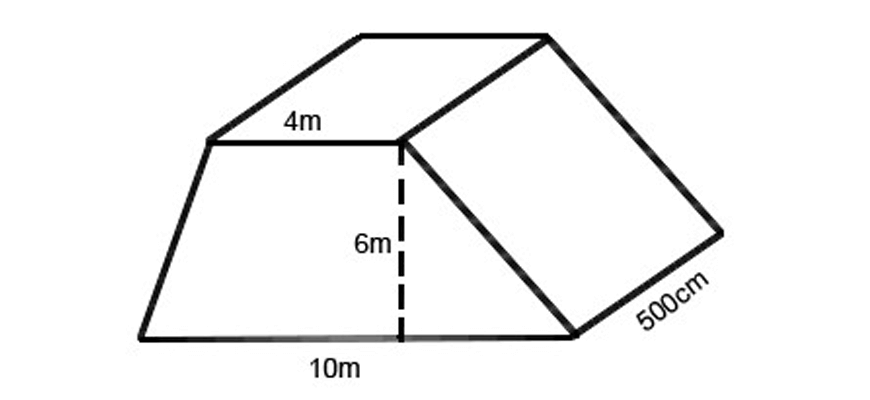### Solution:

To find the volume of this solid, we must first determine the area of the base of the prism. In this question, the base is a trapezium (as shown below):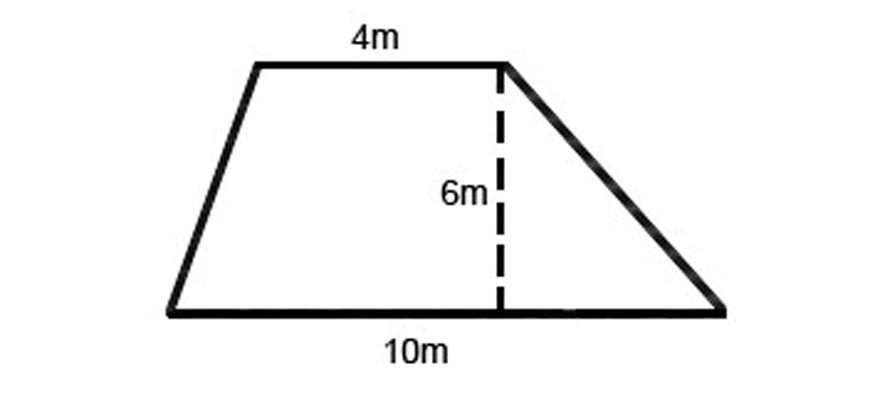Therefore,

$$A_{\text{base}} = \frac{1}{2}(4+10) \times = 42 m^{2}$$

We then must multiply this value for $$A_{\text{base}}$$ with the value for the height of the prism. In this question, the height is 500 cm (or 5 metres).

∴$$V_{\text{prism}}=A_{\text{base}} ×h=(42)(5)=210 m^3$$

Note to students:

Remember to convert all units of lengths in the question to a single unit so that you are only working with one!

## Volumes of pyramid and cone:

A pyramid is defined as a solid geometric figure that has a polygon as its base, and triangles as it sides. These triangular sides meed at a singular point called the vertex.

A cone is a more general form of a pyramid which consists of a flat base (most commonly, but not necessarily, circular), which narrows to a singular point called the vertex.

To determine the volume of a cone or a pyramid, we use the exact same formula for both:

$$V_{\text{cone / pyramid}}=\frac{1}{3}×A_{\text{base}} ×h$$

Where:

• $$V_{\text{cone}}$$ is the volume of the pyramid/cone
• $$A_{\text{base}}$$ is the area of the base of the pyramid/cone
• $$h$$ is the perpendicular distance between the vertex of the cone/pyramid and its base.

The most common pyramids and cones you will come across are shown below. Their bases have been shaded in and their perpendicular heights marked.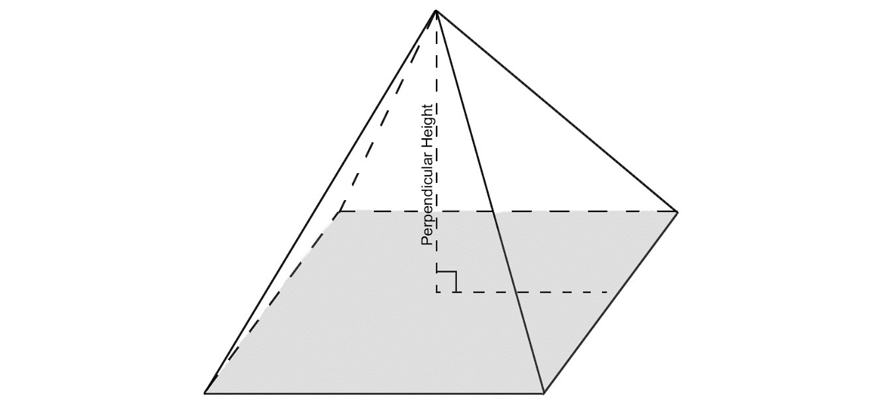Square pyramid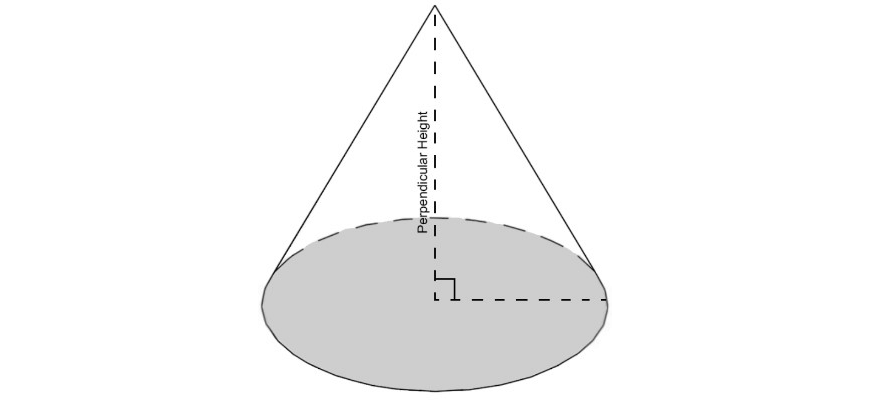Cone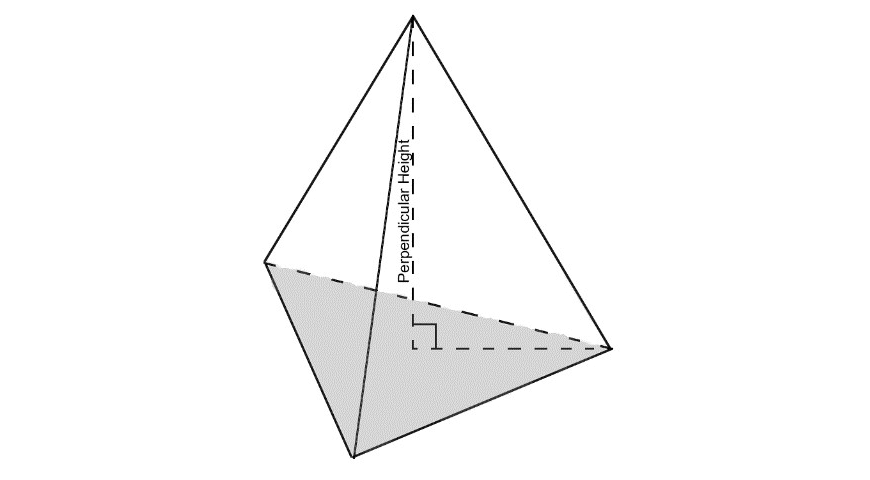Triangular pyramid

Note to students:

The perpendicular height will not always be given to you and you may need to use Pythagoras’ Theorem to calculate it!

### Example:

Determine the volume of the square pyramid below. The diagram is not to scale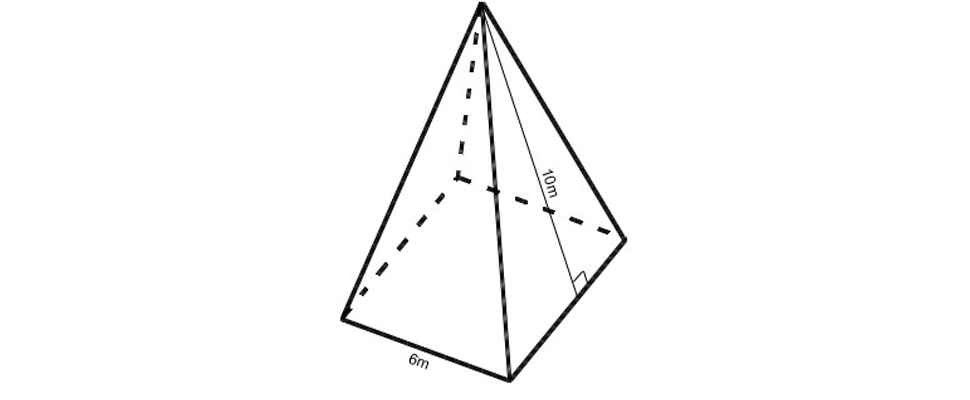### Solution:

To determine the volume of this solid, we must first find the area of its base. As the base is a square, we know that its area is:

$$A_{\text{base}}=(6)(6)=36 m^2$$

We must then find the perpendicular height of the pyramid.

In this question, we are only given the slant height. This slant height is the hypotenuse of the right angled triangle formed by the perpendicular height and half the base length.

So, we must use Pythagoras’ Theorem to determine the perpendicular height: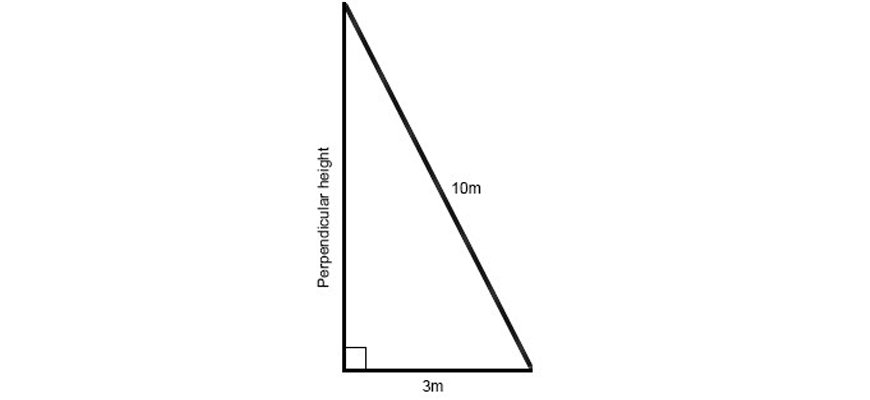∴$$h= \sqrt{10^{2}-3^{2}} \text{(Using Pythagoras’ Theorem)}\\ =\sqrt{100-9}\\ =\sqrt{91} m$$

Hence, we can now determine the volume:

$$V=\frac{1}{3}×A_{\text{base}} ×h \\ =\frac{1}{3}×36×\sqrt{91} \\ =12\sqrt{91} \\ =114.47 m^3 (2 \text{d.p.})$$

### Volume of sphere:

A sphere is defined as a solid geometric figure where every point on its surface is equidistant to a singular point called the centre.

The distance between the centre and any point on the surface of the sphere is referred to as its radius.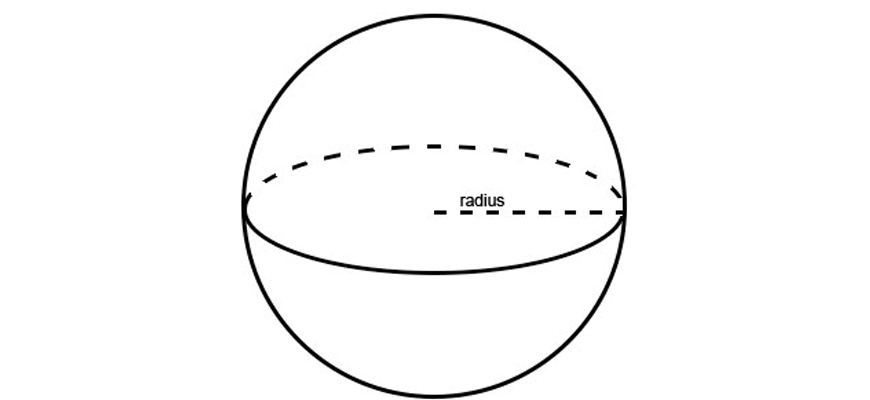Sphere Determine the volume of the triangular prism below: Determine the volume of the triangular prism below:

To find the volume of a sphere, we use the formula:

$$V_{\text{sphere}}=\frac{4}{3} \pi r^3$$

Where:

• $$V_{\text{sphere}}$$ is the volume of the sphere
• $$r$$ is the radius of the sphere

### Example:

Determine the volume of the hemisphere below. The diagram is not to scale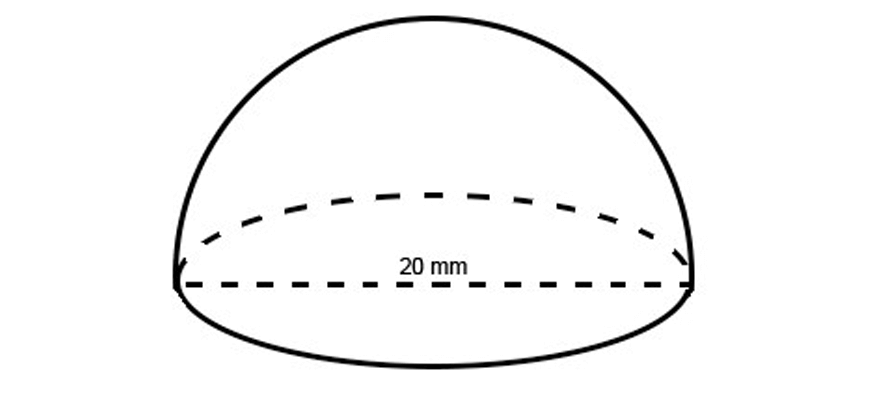Solution:

To determine the volume of this solid, we must first find its radius.

In this question, we are given the diameter of the hemisphere as being 20 mm, meaning that its radius is 10 mm. We also know that a hemisphere has half the volume of its corresponding sphere.

Hence, we can find the volume with the equation:

$$V_{\text{hemisphere}}=\frac{1}{2} V_{\text{sphere}} \\ =(\frac{1}{2})(\frac{4}{3}) \pi ×(10)^3 \\ =(\frac{2000}{3}) \pi \ mm^3$$

## Summary:

To determine the volume of a given solid, first determine whether it is a prism, pyramid, cone, sphere or a combination of them.

Then, find the required values (radius, height, area of the base etc.) to substitute into the given formulas to find the volume of the solid.

$$V_{\text{prism}}=A_{\text{base}} ×h$$
$$V_{\text{cone/pyramid}}=\frac{1}{3}×A_{\text{base}} ×h$$
$$V_{\text{sphere}}=\frac{4}{3} \pi r^3$$

## Year 9 volumes worksheet

Check your skills with the following challenging exercises.

### Question

1. Determine the volume of the triangular prism below: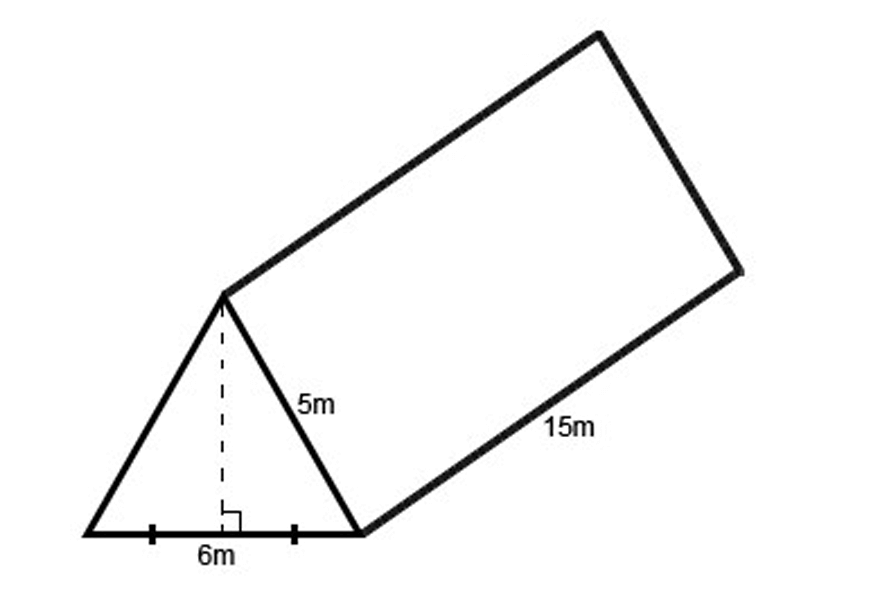2. Find the volume of the prism: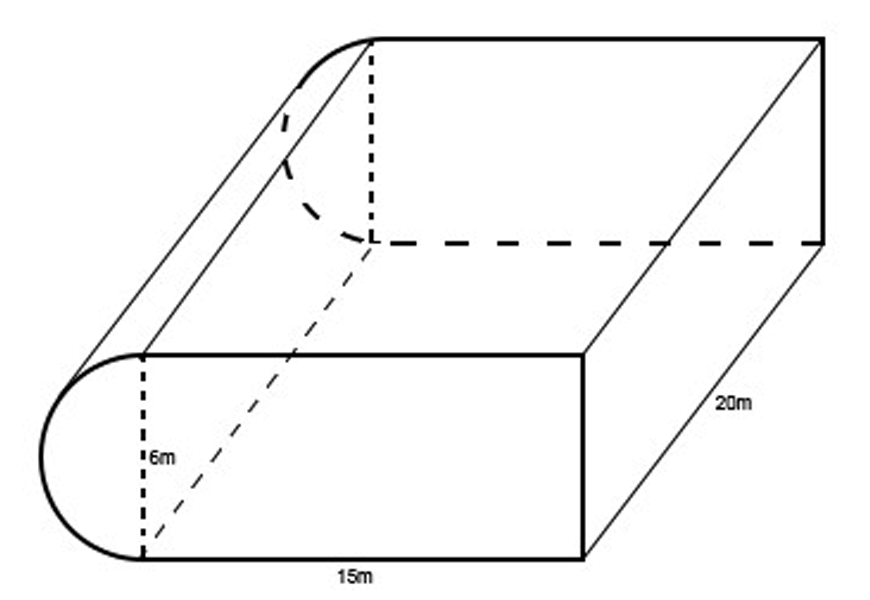3. Determine the volume of the below solid: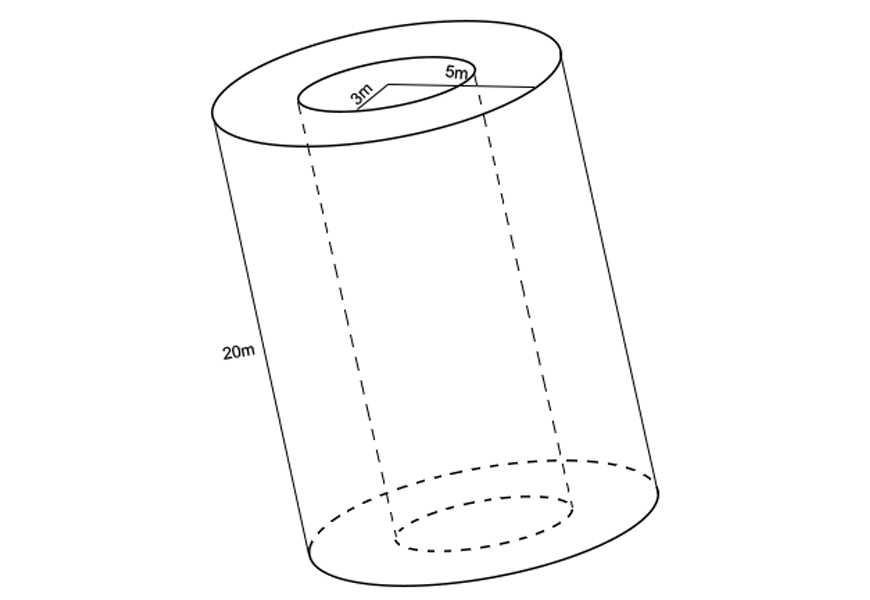4. What is the radius of a sphere with volume $$\frac{256}{3} \pi cm^3$$?

5. Determine the height of the composite solid below given that its volume is $$240 m^2$$: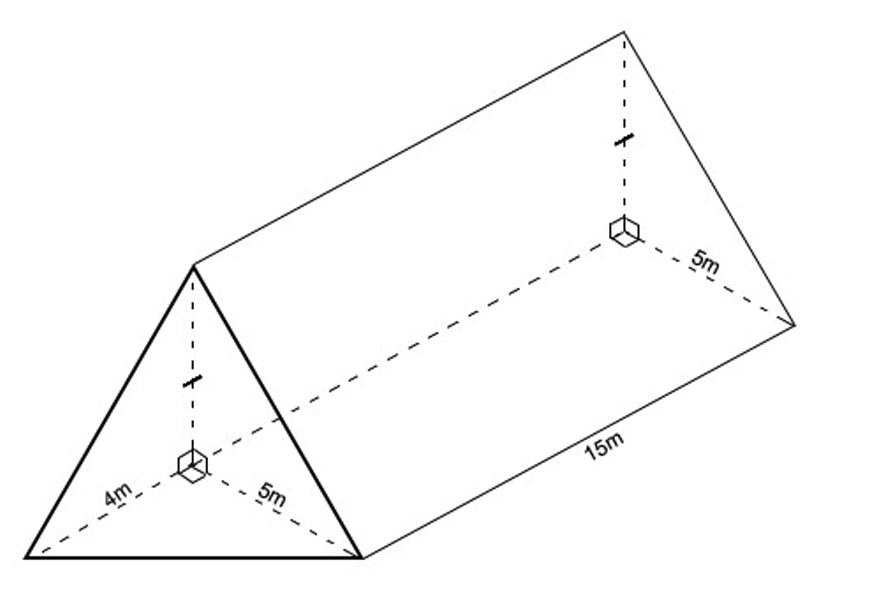6. Find the volume of the below prism: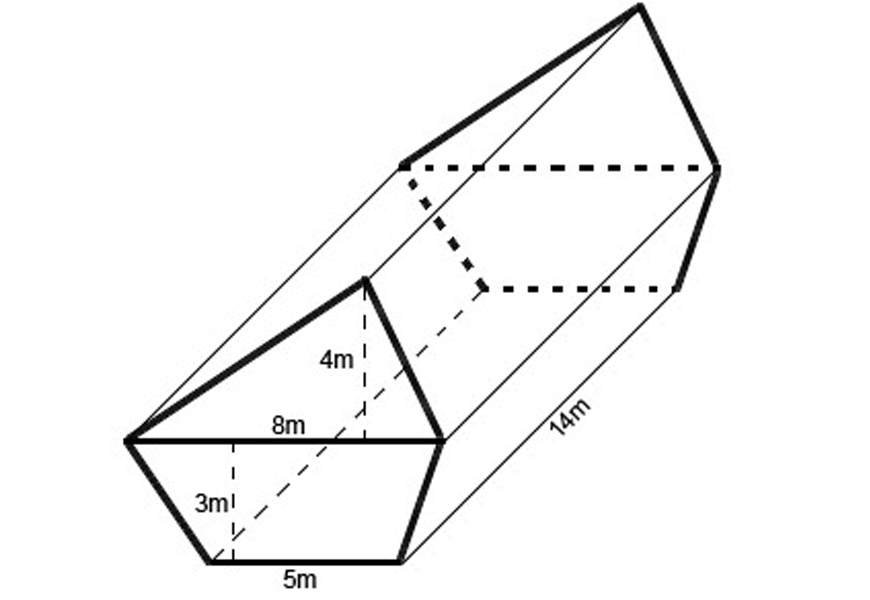7. A builder wants to construct a garden feature made of cement. If the cost of cement is \$50 per cubic metre, determine its cost: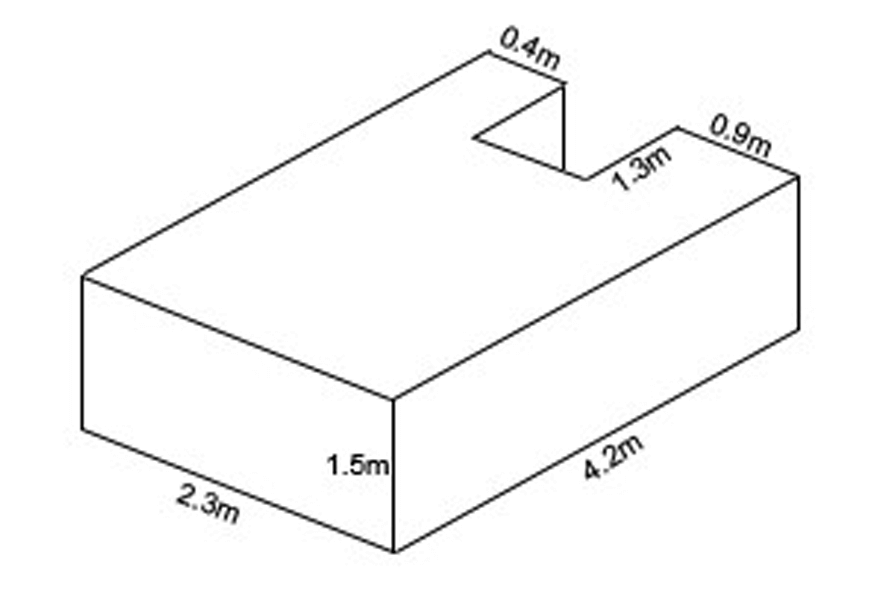8. Find the volume of the solid shown below: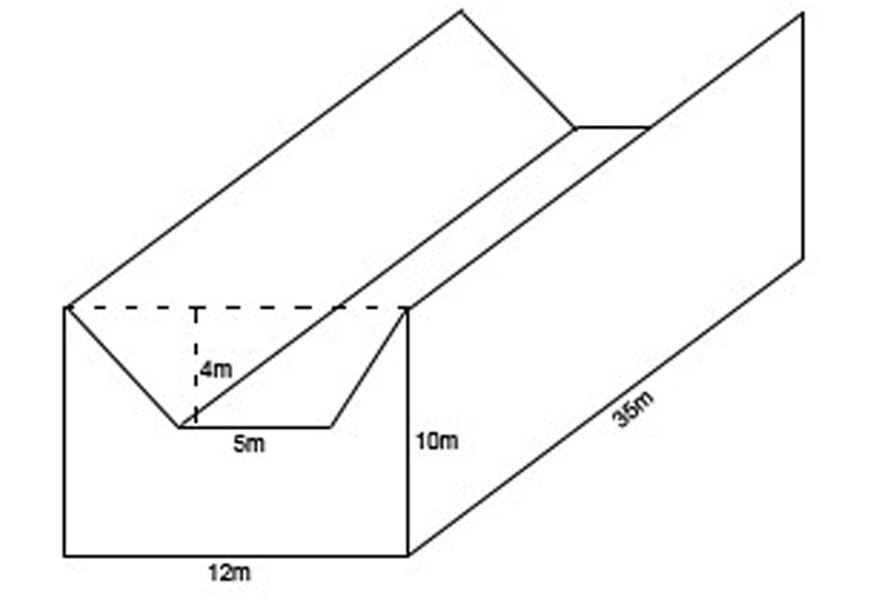9. Find the value of $$x$$ given that the volume of this trapezoidal prism is $$264 m^3$$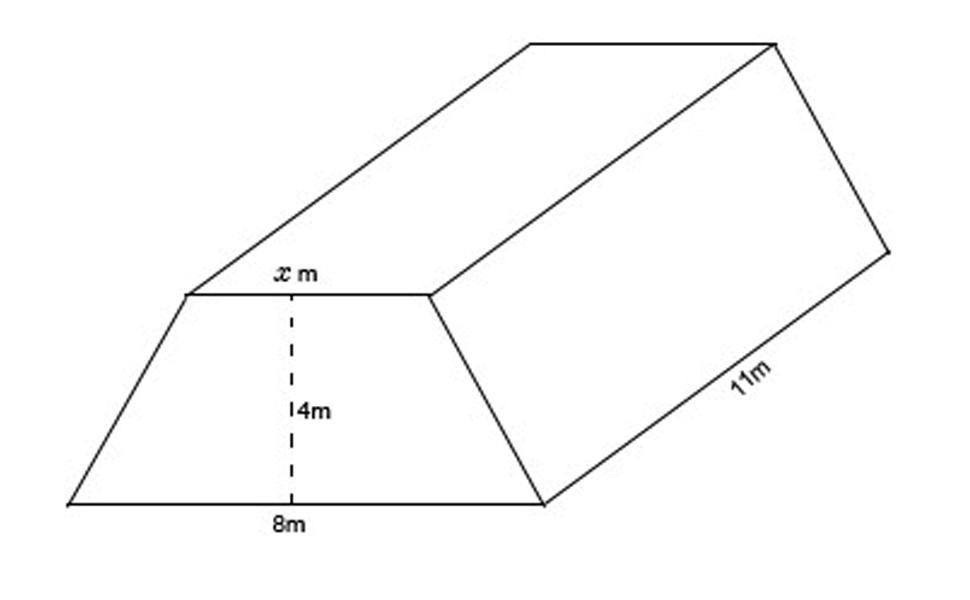10. Find the value  $$r$$ for given that the volume of this composite solid is $$36 \pi m^3$$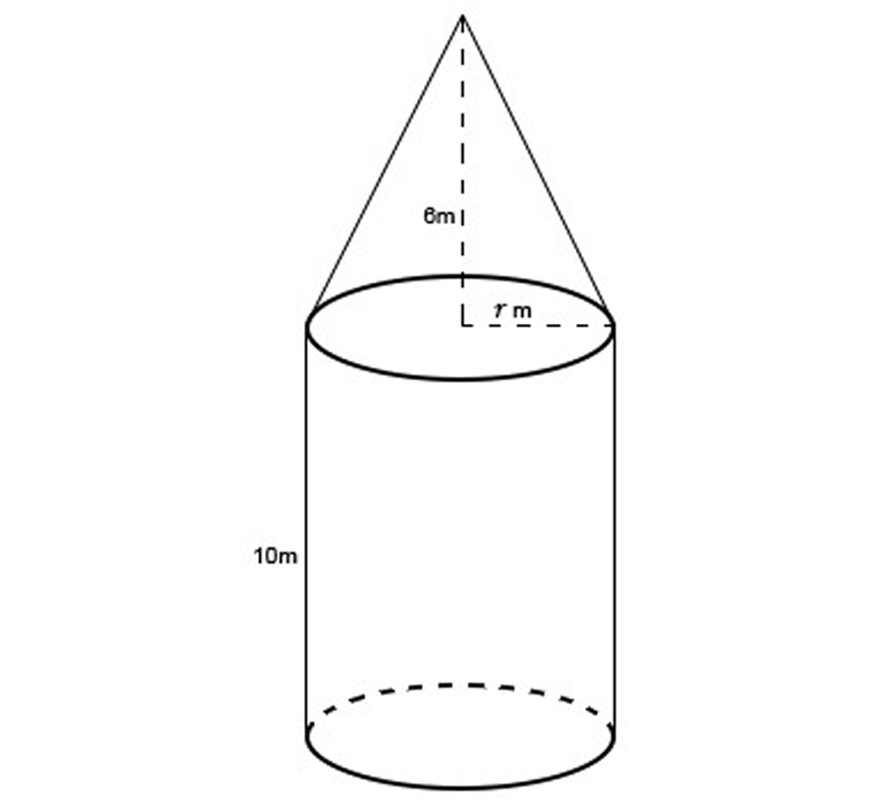### Worked Solutions:

1. Using Pythagoras’ theorem to determine the height of the triangle:

$$h_{\text{triangle}}=\sqrt{5^2-3^2} \\ =4 m \\ ∴A_{\text{base}}=\frac{1}{2} bh_{\text{triangle}} \\ =\frac{1}{2} (6)(4) \\ =12 m^2 \\ ∴V=A_{\text{base}}×h \\ =(12)(15) \\ =180 m^3 \\$$

2. Determining the area of the prism’s base:

$$A_{\text{base}}=A_{\text{semicircle}}+A_{\text{rectangle}} \\ =\frac{1}{2} \pi (3)^2+6×15 \\ =104.13 m^2 (\text{2d.p})\\ ∴V=A_{\text{base}} \times h=(104.13…)(20)\\ =2082.74…m^3 \\$$

3. The solid shown is a prism:

$$A_{\text{base}}=A_{\text{large circle}}-A_{\text{smaller circle}} \\ = \pi (5)^2- \pi (3)^2 \\ =16 \pi m^2 \\ ∴V=A_{\text{base}} \times h \\ =(16 \pi)(20) \\ =320 \pi \\ = 1005.31 m^3 (\text{2d.p}) \\$$

4.

$$V_{\text{sphere}}=\frac{4}{3} \pi r^3 ≜\frac{256}{3} \pi \\ r^3=64 \\ ∴r=4 \text{cm} \\$$

5. The composite solid in this question is comprised of a triangular pyramid and a triangular prism. Let $$x$$  be the height of the composite solid.

\begin{align*}
V_{\text{pyramid}}= & \ \frac{1}{3} × A_{\text{base}} × h \\
= & \ \frac{1}{3} × [\frac{1}{2} (4)(5)] × (x) \\
= & \ \frac{10}{3}x \\
V_{\text{prism}}= & \ A_{\text{base}} ×h \\
= & \ \frac{1}{2} (5)(x) × 15 \\
= & \ \frac{75}{2}x \\
∵V_{\text{solid}}= & \ 240 \\
\frac{75}{2} x+ \frac{10}{3} x= & \ 240 \\
\frac {245}{6} x = & \ 240 \\
x= & \ \frac{288}{49} \\
= & \ 5.87 m (\text{2d.p.})\\
\end{align*}

6.

$$A_{\text{base}}=A_{\text{triangle}}+A_{\text{trapezium}} \\ =\frac{1}{2} (4)(8)+ \frac{1}{2} (8+5)(3) \\ =\frac{71}{2} m^2 \\ ∴V=A_base×h \\ =(71/2)(14) \\ =497 m^3 \\$$

7.

$$A_{\text{base}}=(4.2)(2.3)-(1)(1.3) \\ =8.36 m^2 \\ ∴V=A_{\text{base}} \times h \\ =(8.36)(1.5) \\ =12.54 m^3 \\ ∴ \text{Cost}=(12.54)×50 \\ =627$$

8. The solid shown is a prism:

$$A_{\text{base}}=A_{\text{rectangle}}-A_{\text{trapezium}} \\ =(12)(10)-\frac{1}{2} (5+12)(4) \\ =86 m^2 \\ ∴V=A_{\text{base}} \times h \\ =(86)(35) \\ =3010 m^3 \\$$

9.

$$A_{\text{base}}=\frac{1}{2} (x+8)(4) \\ =2(x+8) \\ =(2x+16) m^2 \\ V=A_{\text{base}} \times h \\ =(2x+16)(11)≜264 \\ ∴2x+16=24 \\ 2x=8 \\ x=4 m \\$$

10. The composite solid is comprise of a cone and a cylinder:

$$V_{\text{solid}}=V_{\text{cone}}+V_{\text{cylinder}} \\ =\frac{1}{3} \times \pi r^2 (6)+ \pi r^2 (10) \\ =12 \pi r^2 \\ ∵V_{\text{solid}}=36 \pi \\ ∴12 \pi r^2=36 \pi \\ r^2=3 \\ r=\sqrt{3} m \\$$

## Need help with trigonometry?

In the next article, we get stuck into trigonometry and its applications.

© Matrix Education and www.matrix.edu.au, 2021. Unauthorised use and/or duplication of this material without express and written permission from this site’s author and/or owner is strictly prohibited. Excerpts and links may be used, provided that full and clear credit is given to Matrix Education and www.matrix.edu.au with appropriate and specific direction to the original content.

### Get free study tips and resources delivered to your inbox.

Join 75,893 students who already have a head start.

Our website uses cookies to provide you with a better browsing experience. If you continue to use this site, you consent to our use of cookies. Read our cookies statement.